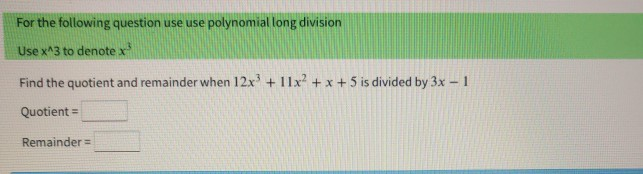Home / Answered Questions / Other / for-the-following-question-use-use-polynomial-long-division-use-x-3-to-denotex-find-the-quotient-and-aw926

# (Solved): For The Following Question Use Use Polynomial Long Division Use X^3 To Denotex Find The Quotient And...can you break down this for me please

For the following question use use polynomial long division Use x^3 to denotex Find the quotient and remainder when 12x + 11xÂ² + x + 5 is divided by 3x - 1 Quotient = Remainder

We have an Answer from Expert